﻿ 复合材料螺旋桨强度评估方法研究
 舰船科学技术2016, Vol. 38Issue (5): 30-34,38PDF

Reaearch on the evaluation method of propeller-strength composite materials
YANG Wen-zhi, ZHU Xi, CHEN Yue, PEI Qiu-qiu
Department of Naval Architecture Engineering, Naval University of Engineering, Wuhan 430033, China
Abstract: In order to realize an accurate assessment of the strength of the propeller, firstly, the strength of the assessment about propeller of specific overlay under hydrodynamic loads was conducted,which was based on the Hashin strength criterion.Then, given that the simulation of propeller under hydrodynamic load was of great limitation, the equivalent concentrated force was studied. Lastly, the static strength experiment shows the finite element calculation results have good agreement with the test results. In addition, the strain of every measuring point increased linearlywhich effectively supported the reliability of the strength.
Key words: composite     propeller     strength evaluation
0 引 言

1 复合材料螺旋桨强度分析 1.1 有限元模型及铺层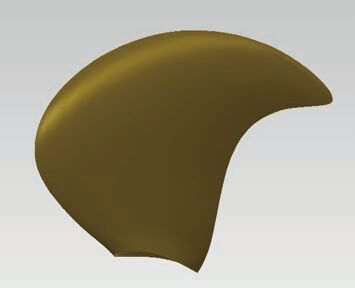图 1 桨叶几何模型 Fig. 1 Blade geometry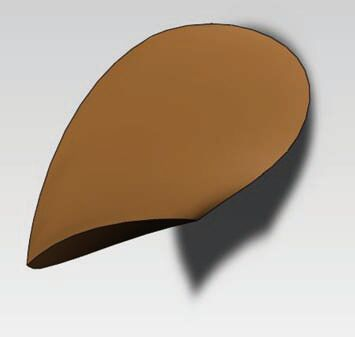图 2 芯材几何模型 Fig. 2 Core geometry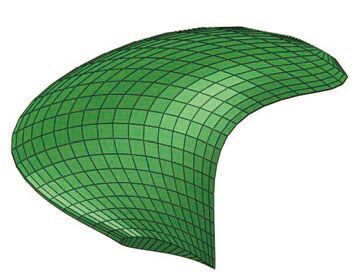图 3 有限元网格 Fig. 3 Finite element mesh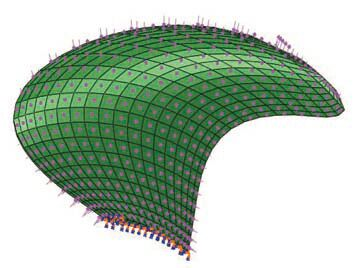图 4 载荷与边界条件 Fig. 4 Loads and boundary conditions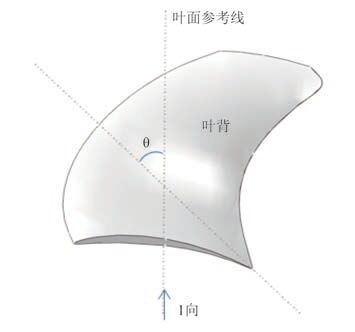图 5 铺层示意图 Fig. 5 Overlay schematic diagram
1.2 Hashin 失效准则

Hashin 失效准则不仅给出了材料失效的条件，而且给出了材料失效的模式。二维 Hashin 失效准则的形式如下：

1）纤维拉伸失效（${\sigma _{{\rm{11}}}}>0$）

 ${{\left( \frac{{{\sigma }_{\text{11}}}}{{{X}_{T}}} \right)}^{\text{2}}}+\alpha {{\left( \frac{{{\tau }_{\text{12}}}}{{{S}_{L}}} \right)}^{\text{2}}}=\text{1};$

2）纤维压缩失效（${\sigma _{{\rm{11}}}} < 0$）

 $\left( \frac{{{\sigma }_{\text{11}}}}{{{X}_{C}}} \right)=\text{1};$

3）基体拉伸失效（${\sigma _{{\rm{22}}}}>0$）

 ${{\left( \frac{{{\sigma }_{\text{22}}}}{{{Y}_{T}}} \right)}^{\text{2}}}+\alpha {{\left( \frac{{{\tau }_{\text{12}}}}{{{S}_{L}}} \right)}^{\text{2}}}=\text{1};$

4）基体压缩失效（${\sigma _{{\rm{22}}}} < 0$）

 ${{\left( \frac{{{\sigma }_{\text{22}}}}{\text{2}{{S}_{T}}} \right)}^{\text{2}}}+\left[ {{\left( \frac{{{Y}_{C}}}{\text{2}{{S}_{T}}} \right)}^{\text{2}}}-\text{1} \right]\frac{{{\sigma }_{\text{22}}}}{\text{2}{{Y}_{C}}}+{{\left( \frac{{{\tau }_{\text{12}}}}{{{S}_{L}}} \right)}^{\text{2}}}=\text{1}。$

1.3 校核结果分析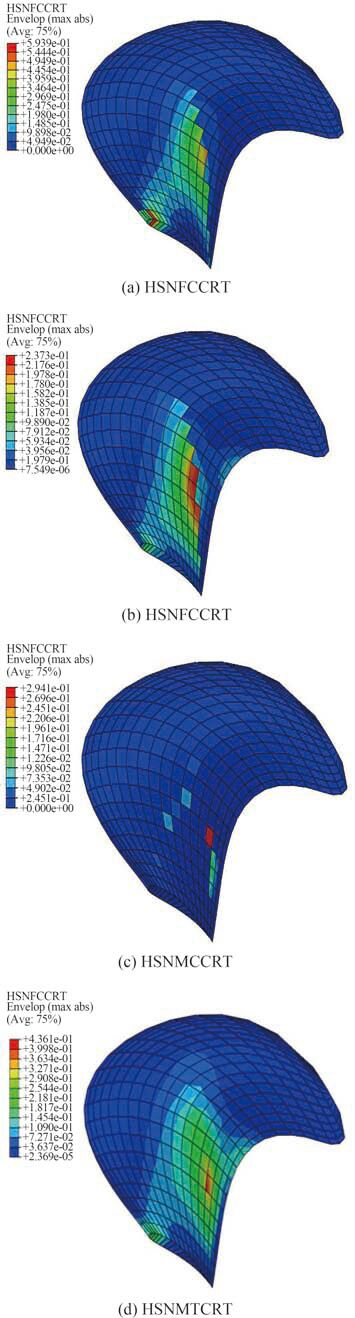图 6 目标桨失效模式比较 Fig. 6 Comparison of target propeller failure modes
2 等效集中力研究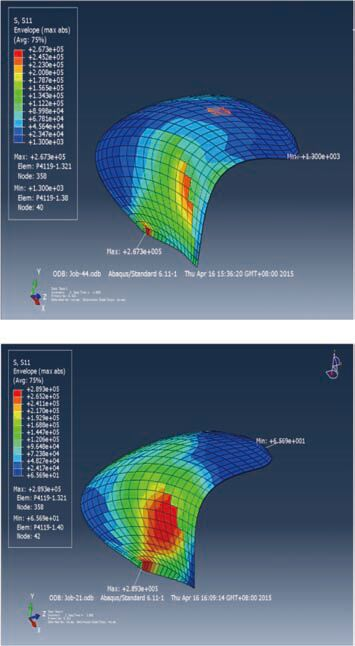图 7 两种载荷应力对比 Fig. 7 Comparison of the two load
3 模型桨成型工艺与静强度试验 3.1 模型桨成型工艺

3.2 测点布置及加载方式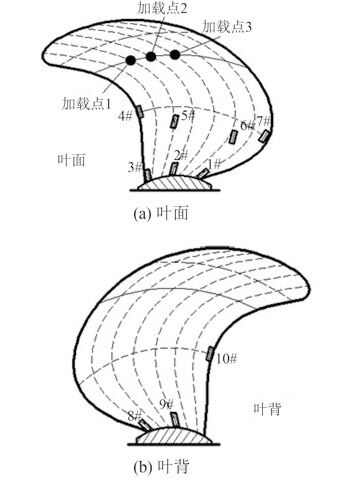图 8 测点布置示意图 Fig. 8 Measuring points layout diagram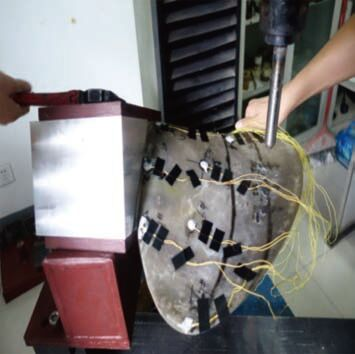图 9 试验实施过程 Fig. 9 The test process

3.3 试验结果与仿真结果对比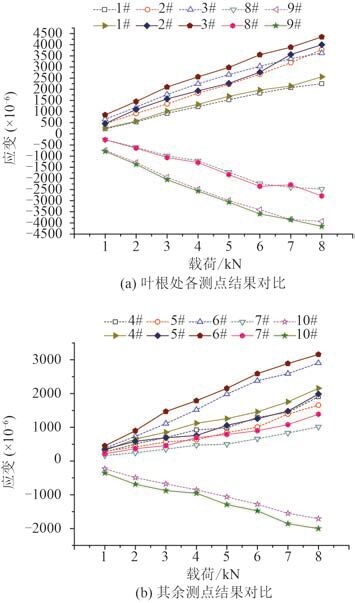图 10 各测点的试验值与有限元结果对比 Fig. 10 Comparison of experimental and calculated results

4 结语

1）夹芯结构复合材料螺旋桨在水动力载荷作用下应重点关注桨叶根部以及夹芯泡沫与纤维布交接处的强度。

2）本文对等效集中力原则进行了初步探究，认为可通过关键强度区域应力等效原则将设计工况下水动力载荷等效为集中力载荷，从而可以达到简化试验的目的。

3）强度试验结果表明，有限元计算所得的各测点对应的应变分量均与试验值所测得的各测点对应的应变量吻合较好，各曲线的分布趋势基本一致，当最大载荷加载到 8 kN 时各测点应变均在弹性范围内，桨叶未发生破坏，充分验证了基于 Hashin 强度准则对桨叶强度进行评估的可靠性。

  张帅, 朱锡, 孙海涛, 等. 船用复合材料螺旋桨研究进展[J]. 力学进展 , 2012, 42 (5) :620–633. ZHANG Shuai, ZHU Xi, SUN Hai-tao, et al. Review of re-searches on composite marine propellers[J]. Advances in Mechanics , 2012, 42 (5) :620–633.  张远双. 螺旋桨强度计算的参数化设计[J]. 武汉船舶职业技术学院学报 , 2011 (5) :18–20. ZHANG Yuan-shuang. Research of propeller's strength calculation by parameterized method[J]. Journal of Wuhan Institute of Shipbuilding Technology , 2011 (5) :18–20.  王玉华. 大侧斜螺旋桨强度研究[J]. 船舶力学 , 1998, 2 (2) :44–51. WANG Yu-hua. Comparative studies on highly-Skewed pro-peller strength[J]. Journal of Ship Mechanics , 1998, 2 (2) :44–51.  朱骏飞.导管螺旋桨水动力分析与优化研究[D].武汉:武汉理工大学, 2013. ZHU Jun-fei. Research on hydrodynamic analysis and optimization of duct propeller[D]. Wuhan:Wuhan University of Technology, 2013. http://cdmd.cnki.com.cn/article/cdmd-10497-1014163511.htm  刘竹青, 陈奕宏, 姚志崇. 基于面元法及有限元法耦合的螺旋桨强度计算[J]. 中国造船 , 2012, 53 (S1) :25–30. LIU Zhu-qing, CHEN Yi-hong, YAO Zhi-chong. Static strength analysis of civil ship propellers[J]. Shipbuilding of China , 2012, 53 (S1) :25–30.  LIN H J. Strength evaluation of a composite marine propeller blade[J]. Journal of Reinforced Plastics and Composites , 2005, 24 (17) :1791–1807. DOI:10.1177/0731684405052199  BLASQUES J P, BERGGREEN C, ANDERSEN P. Hydro-elastic analysis and optimization of a composite marine pro-peller[J]. Marine Structures , 2010, 23 (1) :22–38. DOI:10.1016/j.marstruc.2009.10.002